# 5.5 Violations of the Octet Rule

$$\newcommand{\vecs}{\overset { \rightharpoonup} {\mathbf{#1}} }$$ $$\newcommand{\vecd}{\overset{-\!-\!\rightharpoonup}{\vphantom{a}\smash {#1}}}$$$$\newcommand{\id}{\mathrm{id}}$$ $$\newcommand{\Span}{\mathrm{span}}$$ $$\newcommand{\kernel}{\mathrm{null}\,}$$ $$\newcommand{\range}{\mathrm{range}\,}$$ $$\newcommand{\RealPart}{\mathrm{Re}}$$ $$\newcommand{\ImaginaryPart}{\mathrm{Im}}$$ $$\newcommand{\Argument}{\mathrm{Arg}}$$ $$\newcommand{\norm}{\| #1 \|}$$ $$\newcommand{\inner}{\langle #1, #2 \rangle}$$ $$\newcommand{\Span}{\mathrm{span}}$$ $$\newcommand{\id}{\mathrm{id}}$$ $$\newcommand{\Span}{\mathrm{span}}$$ $$\newcommand{\kernel}{\mathrm{null}\,}$$ $$\newcommand{\range}{\mathrm{range}\,}$$ $$\newcommand{\RealPart}{\mathrm{Re}}$$ $$\newcommand{\ImaginaryPart}{\mathrm{Im}}$$ $$\newcommand{\Argument}{\mathrm{Arg}}$$ $$\newcommand{\norm}{\| #1 \|}$$ $$\newcommand{\inner}{\langle #1, #2 \rangle}$$ $$\newcommand{\Span}{\mathrm{span}}$$$$\newcommand{\AA}{\unicode[.8,0]{x212B}}$$

Three cases can be constructed that do not follow the Octet Rule, and as such, they are known as the exceptions to the Octet Rule. Following the Octet Rule for Lewis Dot Structures leads to the most accurate depictions of stable molecular and atomic structures and because of this we always want to use the octet rule when drawing Lewis Dot Structures. However, it is hard to imagine that one rule could be followed by all molecules. There is always an exception, and in this case, three exceptions. The Octet Rule is violated in these three scenarios:

1. When there are an odd number of valence electrons
2. When there are too few valence electrons
3. When there are too many valence electrons

Reminder: Always use the Octet Rule when drawing Lewis Dot Structures, these exceptions will only occur when necessary.

## Exception 1: Species with Odd Numbers of Electrons

The first exception to the Octet Rule is when there are an odd number of valence electrons. An example of this would be the nitrogen (II) oxide molecule ($$NO$$). Nitrogen atom has 5 valence electrons while the oxygen atom has 6 electrons. The total would be 11 valence electrons to be used. The Octet Rule for this molecule is fulfilled in the above example, however that is with 10 valence electrons. The last one does not know where to go. The lone electron is called an unpaired electron. But where should the unpaired electron go? The unpaired electron is usually placed in the Lewis Dot Structure so that each element in the structure will have the lowest formal charge possible. The formal charge is the perceived charge on an individual atom in a molecule when atoms do not contribute equal numbers of electrons to the bonds they participate in. The formula to find a formal charge is:

Formal Charge= [# of valence e- the atom would have on its own] - [# of lone pair electrons on that atom] - [# of bonds that atom participates in]

No formal charge at all is the most ideal situation. An example of a stable molecule with an odd number of valence electrons would be nitrogen monoxide. Nitrogen monoxide has 11 valence electrons (Figure 1). If you need more information about formal charges, see Lewis Structures. If we were to consider the nitrogen monoxide cation ($$NO^+$$ with ten valence electrons, then the following Lewis structure would be constructed:Figure 1. Lewis dot structure for the $$NO^+$$ ion with ten valence electrons.

Nitrogen normally has five valence electrons. In Figure 1, it has two lone pair electrons and it participates in two bonds (a double bond) with oxygen. This results in nitrogen having a formal charge of +1. Oxygen normally has six valence electrons. In Figure 1, oxygen has four lone pair electrons and it participates in two bonds with nitrogen. Oxygen therefore has a formal charge of 0. The overall molecule here has a formal charge of +1 (+1 for nitrogen, 0 for oxygen. +1 + 0 = +1). However, if we add the eleventh electron to nitrogen (because we want the molecule to have the lowest total formal charge), it will bring both the nitrogen and the molecule's overall charges to zero, the most ideal formal charge situation. That is exactly what is done to get the correct Lewis structure for nitrogen monoxide: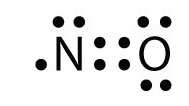Figure 2. The proper Lewis structure for $$NO$$ molecule with 11 valence electrons

There are actually very few stable molecules with odd numbers of electrons that exist, since that unpaired electron is willing to react with other unpaired electrons. Most odd electron species are highly reactive, which we call Free Radicals. Because of their instability, free radicals bond to atoms in which they can take an electron from in order to become stable, making them very chemically reactive. Radicals are found as both reactants and products, but generally react to form more stable molecules as soon as they can. To emphasize the existence of the unpaired electron, radicals are denoted with a dot in front of their chemical symbol as with $${\cdot}OH$$, the hydroxyl radical. An example of a radical you may by familiar with already is the gaseous chlorine atom, denoted $${\cdot}Cl$$. Interestingly, molecules with an odd number of Valence electrons will always be paramagnetic.

## Exception 2: Incomplete Octets

The second exception to the Octet Rule is when there are too few valence electrons that results in an incomplete Octet. There are even more occasions where the octet rule does not give the most correct depiction of a molecule or ion. This is also the case with incomplete octets. Species with incomplete octets are pretty rare and generally are only found in some beryllium, aluminum, and boron compounds including the boron hydrides. Let's take a look at one such hydride, $$BH_3$$ (Borane).

If one was to make a Lewis structure for $$BH_3$$ following the basic strategies for drawing Lewis structures, one would probably come up with this structure (Figure 3):

The problem with this structure is that boron has an incomplete octet; it only has six electrons around it. Hydrogen atoms can naturally only have only 2 electrons in their outermost shell (their version of an octet), and as such there are no spare electrons to form a double bond with boron. One might surmise that the failure of this structure to form complete octets must mean that this bond should be ionic instead of covalent. However, boron has an electronegativity that is very similar to hydrogen, meaning there is likely very little ionic character in the hydrogen to boron bonds, and as such this Lewis structure, though it does not fulfill the octet rule, is likely the best structure possible for depicting BH3 with Lewis theory. One of the things that may account for BH3's incomplete octet is that it is commonly a transitory species, formed temporarily in reactions that involve multiple steps.

Let's take a look at another incomplete octet situation dealing with boron, BF3 (Boron trifluorine). Like with BH3, the initial drawing of a Lewis structure of BF3 will form a structure where boron has only six electrons around it (Figure 4).

If you look Figure 4, you can see that the fluorine atoms possess extra lone pairs that they can use to make additional bonds with boron, and you might think that all you have to do is make one lone pair into a bond and the structure will be correct. If we add one double bond between boron and one of the fluorines we get the following Lewis Structure (Figure 5):

Each fluorine has eight electrons, and the boron atom has eight as well! Each atom has a perfect octet, right? Not so fast. We must examine the formal charges of this structure. The fluorine that shares a double bond with boron has six electrons around it (four from its two lone pairs of electrons and one each from its two bonds with boron). This is one less electron than the number of valence electrons it would have naturally (Group seven elements have seven valence electrons), so it has a formal charge of +1. The two flourines that share single bonds with boron have seven electrons around them (six from their three lone pairs and one from their single bonds with boron). This is the same amount as the number of valence electrons they would have on their own, so they both have a formal charge of zero. Finally, boron has four electrons around it (one from each of its four bonds shared with fluorine). This is one more electron than the number of valence electrons that boron would have on its own, and as such boron has a formal charge of -1.

This structure is supported by the fact that the experimentally determined bond length of the boron to fluorine bonds in BF3 is less than what would be typical for a single bond (see Bond Order and Lengths). However, this structure contradicts one of the major rules of formal charges: Negative formal charges are supposed to be found on the more electronegative atom(s) in a bond, but in the structure depicted in Figure 5, a positive formal charge is found on fluorine, which not only is the most electronegative element in the structure, but the most electronegative element in the entire periodic table ($$\chi=4.0$$). Boron on the other hand, with the much lower electronegativity of 2.0, has the negative formal charge in this structure. This formal charge-electronegativity disagreement makes this double-bonded structure impossible.

However the large electronegativity difference here, as opposed to in BH3, signifies significant polar bonds between boron and fluorine, which means there is a high ionic character to this molecule. This suggests the possibility of a semi-ionic structure such as seen in Figure 6:

None of these three structures is the "correct" structure in this instance. The most "correct" structure is most likely a resonance of all three structures: the one with the incomplete octet (Figure 4), the one with the double bond (Figure 5), and the one with the ionic bond (Figure 6). The most contributing structure is probably the incomplete octet structure (due to Figure 5 being basically impossible and Figure 6 not matching up with the behavior and properties of BF3). As you can see even when other possibilities exist, incomplete octets may best portray a molecular structure.

As a side note, it is important to note that BF3 frequently bonds with a F- ion in order to form BF4- rather than staying as BF3. This structure completes boron's octet and it is more common in nature. This exemplifies the fact that incomplete octets are rare, and other configurations are typically more favorable, including bonding with additional ions as in the case of BF3 .

Example: $$BF_3$$

Draw the Lewis structure for boron trifluoride (BF3).

SOLUTION

1. Add electrons (3*7) + 3 = 24

2. Draw connectivities: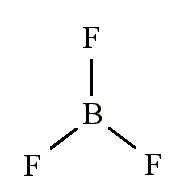3. Add octets to outer atoms:4. Add extra electrons (24-24=0) to central atom:5. Does central electron have octet?

• NO. It has 6 electrons
• Add a multiple bond (double bond) to see if central atom can achieve an octet:6. The central Boron now has an octet (there would be three resonance Lewis structures)

However...

• In this structure with a double bond the fluorine atom is sharing extra electrons with the boron.
• The fluorine would have a '+' partial charge, and the boron a '-' partial charge, this is inconsistent with the electronegativities of fluorine and boron.
• Thus, the structure of BF3, with single bonds, and 6 valence electrons around the central boron is the most likely structure
• BF3 reacts strongly with compounds which have an unshared pair of electrons which can be used to form a bond with the boron: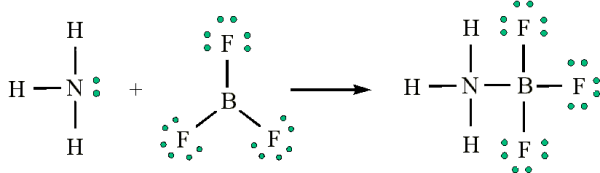## Exception 3: Expanded Valence Shells

More common than incomplete octets are expanded octets where the central atom in a Lewis structure has more than eight electrons in its valence shell. In expanded octets, the central atom can have ten electrons, or even twelve. Molecules with expanded octets involve highly electronegative terminal atoms, and a nonmetal central atom found in the third period or below, which those terminal atoms bond to. For example, $$PCl_5$$ is a legitimate compound (whereas $$NCl_5$$) is not:Expanded valence shells are observed only for elements in period 3 (i.e. n=3) and beyond

The 'octet' rule is based upon available ns and np orbitals for valence electrons (2 electrons in the s orbitals, and 6 in the p orbitals). Beginning with the n=3 principle quantum number, the d orbitals become available (l=2). The orbital diagram for the valence shell of phosphorous is:Hence, the third period elements occasionally exceed the octet rule by using their empty d orbitals to accommodate additional electrons. Size is also an important consideration:

• The larger the central atom, the larger the number of electrons which can surround it
• Expanded valence shells occur most often when the central atom is bonded to small electronegative atoms, such as F, Cl and O.

There is currently much scientific exploration and inquiry into the reason why expanded valence shells are found. The top area of interest is figuring out where the extra pair(s) of electrons are found. Many chemists think that there is not a very large energy difference between the 3p and 3d orbitals, and as such it is plausible for extra electrons to easily fill the 3d orbital when an expanded octet is more favorable than having a complete octet. This matter is still under hot debate, however and there is even debate as to what makes an expanded octet more favorable than a configuration that follows the octet rule.

One of the situations where expanded octet structures are treated as more favorable than Lewis structures that follow the octet rule is when the formal charges in the expanded octet structure are smaller than in a structure that adheres to the octet rule, or when there are less formal charges in the expanded octet than in the structure a structure that adheres to the octet rule.

Example 2: The $$SO_4^{-2}$$ ion

The sulfate ion, SO4-2. is an ion that prefers an expanded octet structure. A strict adherence to the octet rule forms the following Lewis structure:

If we look at the formal charges on this molecule, we can see that all of the oxygen atoms have seven electrons around them (six from the three lone pairs and one from the bond with sulfur). This is one more electron than the number of valence electrons then they would have normally, and as such each of the oxygen atoms in this structure has a formal charge of -1. Sulfur has four electrons around it in this structure (one from each of its four bonds) which is two electrons fewer than the number of valence electrons it would have normally, and as such it carries a formal charge of +2.

If instead we made a structure for the sulfate ion with an expanded octet, it would look like this:

Looking at the formal charges for this structure, the sulfur ion has six electrons around it (one from each of its bonds). This is the same amount as the number of valence electrons it would have naturally. This leaves sulfur with a formal charge of zero. The two oxygens that have double bonds to sulfur have six electrons each around them (four from the two lone pairs and one each from the two bonds with sulfur). This is the same amount of electrons as the number of valence electrons that oxygen atoms have on their own, and as such both of these oxygen atoms have a formal charge of zero. The two oxygens with the single bonds to sulfur have seven electrons around them in this structure (six from the three lone pairs and one from the bond to sulfur). That is one electron more than the number of valence electrons that oxygen would have on its own, and as such those two oxygens carry a formal charge of -1. Remember that with formal charges, the goal is to keep the formal charges (or the difference between the formal charges of each atom) as small as possible. The number of and values of the formal charges on this structure (-1 and 0 (difference of 1) in Figure 12, as opposed to +2 and -1 (difference of 3) in Figure 12) is significantly lower than on the structure that follows the octet rule, and as such an expanded octet is plausible, and even preferred to a normal octet, in this case.

Example 3: The $$ICl_4^-$$ Ion

Draw the Lewis structure for $$ICl_4^-$$ ion.

SOLUTION

1. Count up the valence electrons: 7+(4*7)+1 = 36 electrons

2. Draw the connectivities: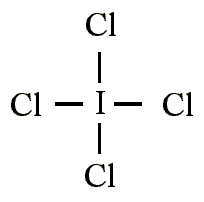3. Add octet of electrons to outer atoms: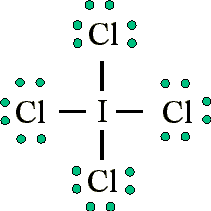4. Add extra electrons (36-32=4) to central atom:5. The ICl4- ion thus has 12 valence electrons around the central Iodine (in the 5d orbitals)Expanded Lewis structures are also plausible depictions of molecules when experimentally determined bond lengths suggest partial double bond characters even when single bonds would already fully fill the octet of the central atom. Despite the cases for expanded octets, as mentioned for incomplete octets, it is important to keep in mind that, in general, the octet rule applies.

## Practice Problems

1. Draw the Lewis structure for the molecule I3-.
2. Draw the molecule ClF3.
3. The central atom for an expanded octet must have an atomic number larger than what?
4. Draw the Lewis structure for the molecule NO2.
5. Which Lewis structure is more likely?or1.2.3. 10 (Sodium and higher)

4.5.## References

1. Petrucci, Ralph H.; Harwood, William S.; Herring, F. G.; Madura, Jeffrey D. General Chemistry: Principles & Modern Applications. 9th Ed. New Jersey. Pearson Education, Inc. 2007.
2. Moore, John W.; Stanitski, Conrad L.; Jurs, Peter C. Chemistry; The Molecular Science. 2nd Ed. 2004.